# Consider the following system at equilibrium. 2CO(g)+O2(g)<--->2CO2(g)

Consider the following system at equilibrium. 2CO(g)+O2(g)<—>2CO2(g)

1. How will increasing the concentration of CO shift the equilibrium?
A) to the right
B) to the left
C) no effect

2. How will increasing the concentration of CO2 shift the equilibrium?
A) to the right
B) to the left
C) no effect

3. How will decreasing the the volume of the container shift the equilibrium?
A) to the right
B) to the left
C) no effect

Concepts and reason
Le Chatlier Principle:
When a change is applied to a system at equilibrium, the equilibrium will shift against the change. The equilibrium of a system will be affected by the changes in temperature, pressure and concentration

Fundamentals
The expression for the equilibrium is given as follows:
For any arbitrary reaction at equilibrium,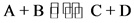The double half arrows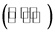in the above reaction indicates that there is a simultaneous change in both directions of the reaction.
Le Châtelier’s principle:
If a system at equilibrium is disturbed, the equilibrium moves in such a way to counteract the change.

The factors that are affecting chemical equilibrium:
oConcentration.
oTemperature.
oPressure (or volume).
Factors that are affecting Equilibrium: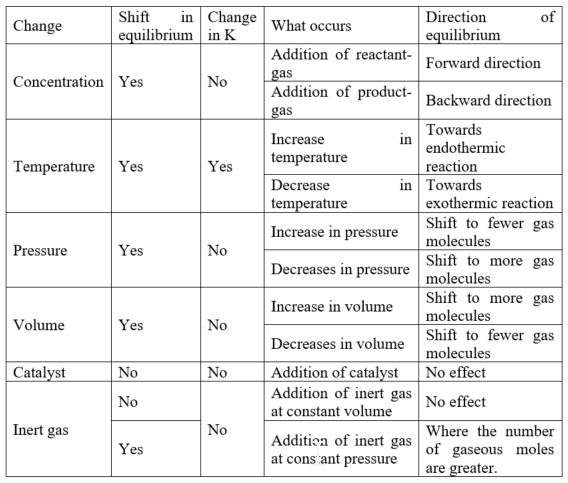Part 1
The given balanced chemical equation is written below.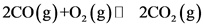The above reaction indicates that carbon monoxide reacts with oxygen and forms carbon dioxide gas.
By increasing the concentration of carbon monoxide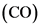, the equilibrium in the reaction shifts towards the right.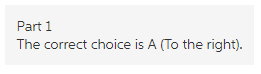Explanation:
The given equilibrium reaction indicates the reaction between carbon monoxide and the oxygen and forms carbon dioxide. In this reaction, by increasing the concentration of the carbon monoxide, the equilibrium shifts towards the right. Since, the reactant concentration increases, the equilibrium stress decreases the concentration of the reactants and therefore, the equilibrium shift towards the right side of the equation.

Part 2
The given balanced chemical equation is written below.The above reaction indicates that carbon monoxide reacts with oxygen and forms carbon dioxide gas.
By increasing the concentration of carbon dioxide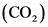, the equilibrium in the reaction shifts towards the left.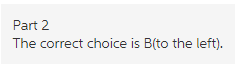Explanation:
The given equilibrium reaction indicates the reaction between carbon monoxide and the oxygen and forms carbon dioxide. In this reaction, by increasing the concentration of the carbon dioxide, the equilibrium shifts towards the left. Since, the product concentration increases, according to Le chattier principle, the equilibrium stress proceeds to decrease the concentration of the products. Therefore, the equilibrium shifts towards the left side of the equation.

Part 3
The given balanced chemical equation is written below.The above reaction indicates that carbon monoxide reacts with oxygen and forms carbon dioxide gas.
By decreasing the volume of the container, the equilibrium shifts towards the right side of the reaction.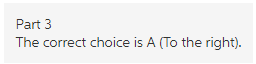Explanation:
The given equilibrium reaction indicates the reaction between carbon monoxide and the oxygen and forms carbon dioxide. In this reaction, by decreasing the volume of the reaction, the equilibrium shifts towards the fewer gas molecule side of the reaction. Since, the volume of the container decreases, the number of moles per unit volume increases and the equilibrium stress will shift to the side with the lesser number of gas molecules. Therefore, the equilibrium shifts towards the right side of the equation. In reactants, three gas molecules are present while in the products, two gas molecules are present.